最丑陋的 C 语言特性：tgmath.h

CIIS 2019丨CIIS 2019丨倒计时10天丨CIIS 2019 嘉宾阵容公布！>>><tgmath.h>是一个在C99引入的，标准C语言库提供的头文件。对于Fortran编写的数值软件，它向C语言提供更加简洁的接口。

2000-08-01 Ulrich Drepper drepper@redhat.com

Joseph S. Myers jsm28@cam.ac.hk

* math/tgmah.h: Make standard compliant. Don’t ask how.

#define cos(X) __tgmath_cos(X)

#define cos(X) __tgmath_cos(X)
#praga compiler_vendor overload __tgmath_cos
double __tgmath_cos (double x)
{return (cos) (x); }
float __tgmath_cos (float x)
{return cosf (x); }
long double __tgmath_cos (long double x)
{return cosl (x); }
...

(简单的习题： 为什么在定义__tgmath_cos(double)时，cos两旁有括号呢？)

glibc的实现必须依靠那些用已经成熟的gcc版本推出的扩展，因此要实现它更加复杂了。

#define cos(X) \
(X is real) ? ( \
(X has type double \
|| X has an integer type) \
? (cos) (X) \
: (X has type long double) \
? cosl(X) \
: cosf (X) \
) : (
(X has type double _Complex) \
? ccos (X)
....

• “x is real”就是sizeof (X) == sizeof (__real__ (X))
• “x has an integer type”就是(typeof (X))1.1 == 1（中等的习题：(__typeof__ (X))0.1 == 0不正确。这是为什么呢？） （事实上，glibc在某些情况使用了__builtin_classify_type，一种嵌入式的内部gcc，而在上述情况使用了另一种相似的替代。）
• “x has type double/long double/float“也能被sizeof区分。但在有些硬件结构下，一些C类型被映射成相同的硬件类型，这时区分的结果可能那么精确，不过在这些硬件结构下这些不同类型的运算都没有差别，而且外部的C语言也不能识别出差别了。就C语言的”as-if”原则来说，这算是相当不错的了。

#define cos(X) ({ result_type __var; \
if (X is real) { \
if ((X has type double) \
|| (X has an integer type)) { \
__var = (cos) (X); \
else if (X has type long double) \
__var = cosl (X); \
...
__var; })

1 ? (int *)0 : (void *)0

1 ? (int *)0 : (void *)1

• 从编译器的角度来说，一个整形常数表达式就是一个整形表达式有一个常数值：编译器能够计算这个常数而不用任何除了常数合并以外的优化。尤其是这个表达式不会用到任何其他变量的值。
• 空指针就是一个值等于整数值0的指针。空指针能够是任何类型的指针。
• 空指针常量是一种句法结构。空指针常量的值在转换成一个指针类型时，是一个空指针（“空指针”和“它的值”都在上文说过了）。空宏展开成空指针。

(其实，当其定义为一个表达式的值时，它就不是一个句法结构了。不过最好就这样假装它是个句法结构，因为大部分情况下，“值为零的整型常量表达式”其实就是字面上的0。)

1 ? (int *)0 : (void *)0

1 ? (int *)0 : (void *)1

1 ? (__typeof__ (X) *)0 : (void *)(X has a integer type)

1 ? (int *)0 : (void *)(!(X has an integer type))

1 ? (__typeof__ (E1))0 : (__typeof__ (E2))0

__typeof__ (*(1 ? (__typeof__ (E1))0 : (__typeof__ (E2))0))

最新评论（18）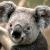引用来自“jQer”的评论

js可称不上优雅，python还差不多。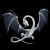引用来自“jQer”的评论

js可称不上优雅，python还差不多。

JavaScript 这门语言相当优雅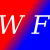引用来自“jQer”的评论

"优雅"应该和具体的语言无关吧，这要看是谁写的程序。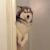引用来自“jQer”的评论

C写出的一看就高深莫测~

+1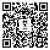引用来自“jQer”的评论

C写出的一看就高深莫测~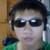引用来自“jQer”的评论

js可称不上优雅，python还差不多。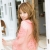引用来自“Jakes”的评论